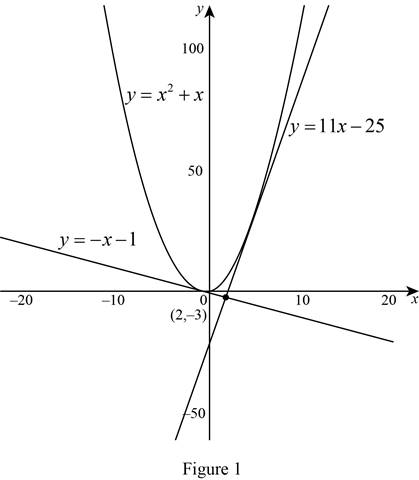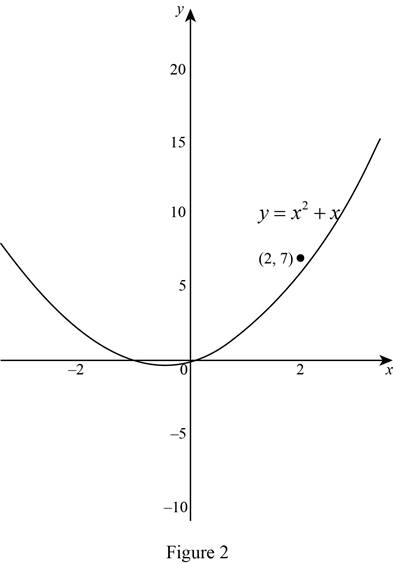# The equation of the tangent lines to the parabola through the points.### Single Variable Calculus: Concepts...

4th Edition
James Stewart
Publisher: Cengage Learning
ISBN: 9781337687805### Single Variable Calculus: Concepts...

4th Edition
James Stewart
Publisher: Cengage Learning
ISBN: 9781337687805

#### Solutions

Chapter 3.1, Problem 58E

(a)

To determine

## To find: The equation of the tangent lines to the parabola through the points.

Expert Solution

The tangent line of curve at (1,0) is y=x1.

The tangent line of curve at (5,30) is y=11x25.

### Explanation of Solution

Given:

The parabola is y=x2+x.

The parabola through the point is (2,3).

Derivative rules:

(1) Power Rule: ddx(xn)=nxn1

(2) Sum Rule: ddx[f(x)+g(x)]=ddx(f(x))+ddx(g(x))

Formula used:

The equation of tangent line at (x1,y1) is, yy1=m(xx1) (1)

Here, m is the slope of the tangent line at (x1,y1) and m=dydx|x=x1.

Calculation:

The derivative of y is dydx, which is obtained as follows.

dydx=ddx(y) =ddx(x2+x)

Apply the sum rule (2).

dydx=ddx(x2)+ddx(x)

Apply the power rule (1) and simplify the expression.

dydx=2x21+1x11=2x+1

Therefore, the derivative of the parabola y=x2+x is 2x+1.

Given parabola is y=x2+x, that is every point of the parabola is (a,a2+a) of the form.

Obtain the equation of the tangent line to the parabola y=x2+x at the point (a,a2+a).

The slope of the tangent line at (a,a2+a) is,

m=dydx|x=a=2(a)+1=2a+1

Thus, the slope of tangent line at (a,a2+a) is m=2a+1 (2)

Note that, the slope of the line passing through the point (x1,x2) and (y1,y2) is m=y2y1x2x1.

The tangent line passes through the point (2,3) and any point (a,a2+a) to the curve is,

m=a2+a(3)a2=a2+a+3a2

Therefore, the slope m=a2+a+3a2 (3)

Compare the equations (2) and (3),

2a+1=a2+a+3a2

Cross multiply the equation and simplify the terms,

(a2)(2a+1)=a2+a+32a2+a4a2=a2+a+3a24a5=0

Solve the above equation and obtain a=1and a=5.

Substitute the values a=1and a=5 in (a,a2+a), the points are (1,0) and (5,30).

Since the slope of the tangent line at (a,a2+a) is m=2a+1, the slope at (1,0) and (5,30) are 1 and 11, respectively.

Substitute (1,0) for (x1,y1) and 1 for m in equation (1),

y0=1(x+1)y=x1

The tangent line to the curve at (1,0) is y=x1.

Substitute (5,30) for (x1,y1) and 11 for m in equation,

y30=11(x5)y30=11x55y=11x55+30y=11x25

The tangent line of curve at (5,30) is y=11x25.

The graph:

The graph of the curve and tangent line as shown below in Figure 1.From Figure 1, it is observed that the lines y=11x25 and y=x1 are tangent to the curve y=x2+x and these two lines are intersect at the point (2,3).

(b)

To determine

Expert Solution

### Explanation of Solution

The given parabola is y=x2+x, that is every point of the parabola is (a,a2+a) of the form.

Note that, the slope of the line passing through the point (x1,x2) and (y1,y2) is m=y2y1x2x1

The tangent line passing through the point (2,7) and any point to the parabola (a,a2+a),

m=a2+a(7)a2=a2+a7a2

Therefore, the slope of the tangent line m=a2+a7a2.

From part (a), the slope of tangent line at (a,a2+a) is, m=2a+1.

Then, the equation becomes,

2a+1=a2+a7a2(2a+1)(a2)=a2+a72a24a+a2=a2+a7a24a+5=0

Note that, the quadratic equation Ax2+Bx+C=0 has no real solution if the discriminant B24AC<0.

B24AC=164(1)(5)=1620=4

Thus, the equation a24a+5=0 has no real solution.

Therefore, it is concluded that there is no line such that the tangent line passing through the point (2,7) to the parabola.

Graph:

The graph of the parabola y=x2+x and the point (2,7) is shown below in Figure 2.From Figure 2, it is observed that there is no tangent line to the parabola through the point (2,7).

### Have a homework question?

Subscribe to bartleby learn! Ask subject matter experts 30 homework questions each month. Plus, you’ll have access to millions of step-by-step textbook answers!